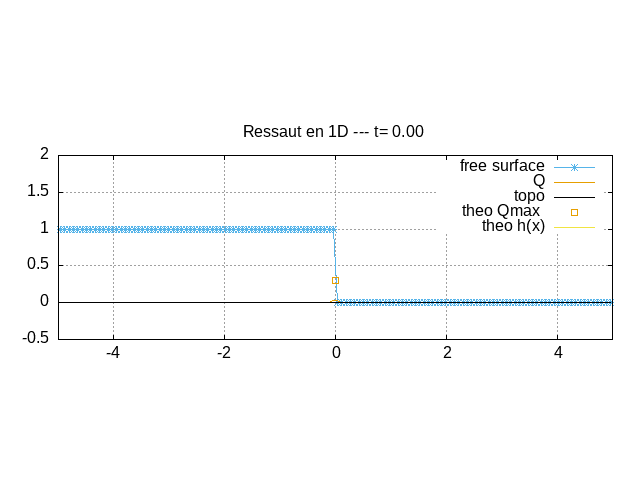# Ideal fluid “dam break problem”,

## Problem

What happens if the dam which retains a lake is suddenly removed? That is the dam break problem.

Problème de la rupture de Barrageanimation of the dam break

## Equations

The classical Shallow Water (Saint Venant) Equations in 1D with no friction. \displaystyle \left\{\begin{array}{l} \partial_t h+\partial_x Q=0\\ \partial_t Q+ \partial_x \left[\dfrac{Q^2}{h}+g\dfrac{h^2}{2}\right] = 0 \end{array}\right. with at t=0, a lake on the left h(|x|<0,t=0)=1 and zero water h(|x|>0,t=0)=0 for x>0. As the flow is not viscous, the convective term is important. The solution is the Ritter 1862 solution with simple waves.

This is solved with http://basilisk.fr/src/saint-venant.h

## Code

#include "grid/cartesian1D.h"
#include "saint-venant.h"

double tmax;

u.n[left]   = neumann(0);
u.n[right]  = neumann(0);

start by a lake on the left and dry on the right, the soil is flat z_b=0

event init (i = 0)
{
foreach(){
zb[] =  0;
h[] =  (x<0)*1  +.0;
u.x[] =0;
}
boundary({zb,h,u});
}

position of domain X0, length L0, no dimension G=1,
run with 512 points (less is not enough)

int main() {
X0 = -5.;
L0 = 10.;
G = 1;
N = 512/4;
tmax=3;

run();
}

Output in gnuplot if the flag gnuX is defined, put in a gifif not

event plot (t<tmax;t+=0.01) {
static FILE * fp = popen ("gnuplot -persist 2> /dev/null", "w");
#ifdef gnuX
#else
if(t==0) fprintf (fp,"set term gif animate;set output 'animate.gif';set size ratio .333333\n");
#endif
fprintf (fp,"\nset grid\n");
fprintf (fp,"set title 'Ressaut en 1D --- t= %.2lf '\n"
"h(x)=(((x-0)<-t)+((x-0)>-t)*(2./3*(1-(x-0)/(2*t)))**2)*(((x-0)<2*t));t= %.2lf  ; "
"p[%g:%g][-.5:2]  '-' u 1:($2+$4) t'free surface' w lp lt 3,"
"'' u 1:($2*$3) t'Q' w l lt 4,\\\n"
"'' u 1:4 t'topo' w l lt -1,\\\n"
"'+' u (0):(0.296296296296296) t'theo Qmax ' , h(x) t 'theo h(x)'\n",
t,t,X0,X0+L0);
foreach()
fprintf (fp,"%g %g %g %g %g\n", x, h[], u.x[], zb[], t);
fprintf (fp,"e\n\n");

if(t==tmax){
fprintf (fp,"! cp animate.gif a2.gif \n");}
}

Output at the end

event end(t=tmax ) {
foreach()
fprintf (stderr,"%g %g %g %g %g\n", x, h[], u.x[], zb[], t);

}

## Run

To compile and run with gnuplot:

  qcc -DgnuX=1   -O2   -o damb damb.c -lm;  ./damb  

## Plots

Plot of the surface and flux at time t=t_{max}: Indeed the analytical solution \displaystyle x<-t, \;\; h(x,t)=1 \displaystyle -t<x<2t, \;\; h(x,t)= (\frac{2}{3}(1-\frac{x}{2t}))^2 \displaystyle 2t < x , \;\; h(x,t)=0 and the numerical one are superposed

set xlabel "x"
h(x)=(((x-0)<-t)+((x-0)>-t)*(2./3*(1-(x-0)/(2*t)))**2)*(((x-0)<2*t))
t=3
p [:][-1:3] 'log' t'free surface' w p lc 3,'' u 1:($3*$2) w l t'Q', '' u 1:4 w l lc -1,h(x) w l lc 1result, free surface (blue) and bottom (black) (script)

## Bibliographie

• Lagrée P-Y “Equations de Saint Venant et application, Ecoulements en milieux naturels” Cours MSF12, M1 UPMC

Version 1: Montpellier 2017